# Quantitative Analysis of Non-Linear High Entropy Economic Systems IV

From: John Conover <john@email.johncon.com>
Subject: Quantitative Analysis of Non-Linear High Entropy Economic Systems IV
Date: 14 Feb 2002 07:38:46 -0000

### Introduction

As mentioned in Section I, Section II and Section III, much of applied economics has to address non-linear high entropy systems-those systems characterized by random fluctuations over time-such as net wealth, equity prices, gross domestic product, industrial markets, etc.

The dynamics of non-linear high entropy systems are probabilistic in nature, and require a suitable methodology for analysis.

### Methodology

The file `ge.jan.02.1962-mar.1.2002` contains the time series of GE's daily close equity price history, from January 2, 1962, through, March 2, 2002, (10,113 days,) inclusive. The data is from Yahoo!'s Historical Prices database, and is adjusted for splits.

The file was converted to a Unix flat file database with the `csv2tsinvest` program:

``````

csv2tsinvest GE ge.csv > ge.jan.02.1962-mar.1.2002

``````

Analysis:

Analyzing the time series for the GE equity price time series:

``````

tsfraction ge.jan.02.1962-mar.1.2002 | tsavg -p
0.000507

tsfraction ge.jan.02.1962-mar.1.2002 | tsrms -p
0.015451

tsgain -p ge.jan.02.1962-mar.1.2002
1.00038773455

``````

where the average of the marginal incrments, ```avg = 0.000507```, the root-mean-square of the marginal increments, `rms = 0.015451`, and the gain of the marginal increments, `g`, and:

``````

avg       0.000507
--- + 1   -------- + 1
rms       0.015451
P = ------- = ------------ = 0.51640670507 .........(1.24)
2           2

``````

are used in the file, `sim`, which contains the single record:

``````

GE, p = 0.51640670507, f = 0.015451

``````

and making the simulation file for GE equity price:

``````

tsinvestsim sim 10113 > ge.jan.02.1962-mar.1.2002.tsinvestsim

``````

where the file, `ge.jan.02.1962-mar.1.2002.tsinvestsim` is the simulated GE equity price time series with identical statistics.

The value of the variables will be used in analysis of the dynamics of GE's equity price, a non-linear high entropy economic system, by comparing the dynamics of the empirical data with the dynamics of both the simulated data and its theoretical abstraction, `constant * erf (1 / sqrt (t))`.

### Example I, GE Equity Price

The file `ge.jan.02.1962-mar.1.2002` contains the time series of GE's daily close equity price history, from January 2, 1962, through, March 2, 2002, (10,113 days,) inclusive. The data is from Yahoo!'s Historical Prices database, and is adjusted for splits.

The file was converted to a Unix flat file database with the `csv2tsinvest` program:

``````

csv2tsinvest GE ge.csv > ge.jan.02.1962-mar.1.2002

``````

The cumulative probability of the duration of the increases and decreases in the average of the marginal increments, `avg`, of GE's equity price:

``````

#!/bin/sh

interval=1

while [ \$interval -le 4048 ]
do

tsmath -l ge.jan.02.1962-mar.1.2002 | tslsq -o | \
tsderivative | tsavgwindow -w \$interval | tsrms -p >> \
ge.jan.02.1962-mar.1.2002.avgwindow

tsmath -l ge.jan.02.1962-mar.1.2002.tsinvestsim | tslsq -o | \
tsderivative | tsavgwindow -w \$interval | tsrms -p >> \
ge.jan.02.1962-mar.1.2002.tsinvestsim.avgwindow

interval=`expr \$interval + 1`

done

``````

which uses the `tsavgwindow` program to calculate the average of the marginal increments over the time interval, `interval`, for all intervals from `1` to `4048`, and the `tsrms` program to calculate the deviation of the average, for GE's equity price.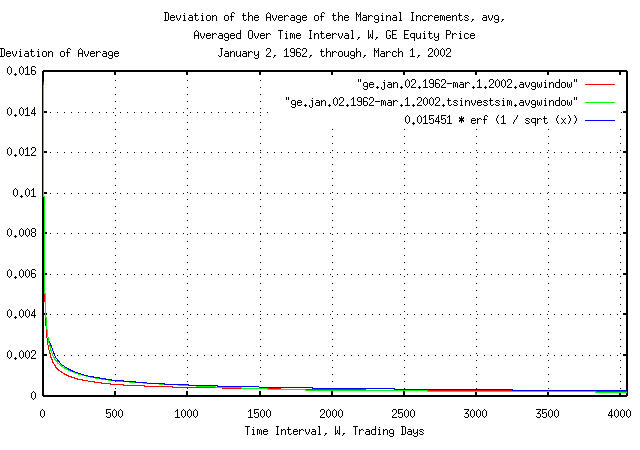Figure I

Figure I is a plot of the cumulative probability of the duration of the increases and decreases in the average of the marginal increments, `avg`, of GE's equity price, and its simulation, with the theoretical value, ```0.015451 * erf (1 / sqrt (t))```. The frequency of the durations of the increases and decreases of up to 4,048 trading days, (about 16 calendar years,) are shown.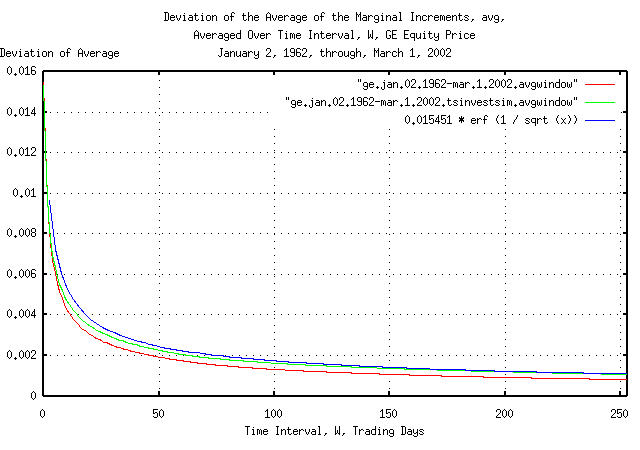Figure II

Figure II is a plot of the cumulative probability of the duration of the increases and decreases in the average of the marginal increments, `avg`, of GE's equity price, and its simulation, with the theoretical value, ```0.015451 * erf (1 / sqrt (t))```. The frequency of the durations of the increases and decreases of up to 253 trading days, (about a calendar year,) are shown. Figure II the same as Figure I, but shows only the frequency of short durations of increases and decreases for clarity.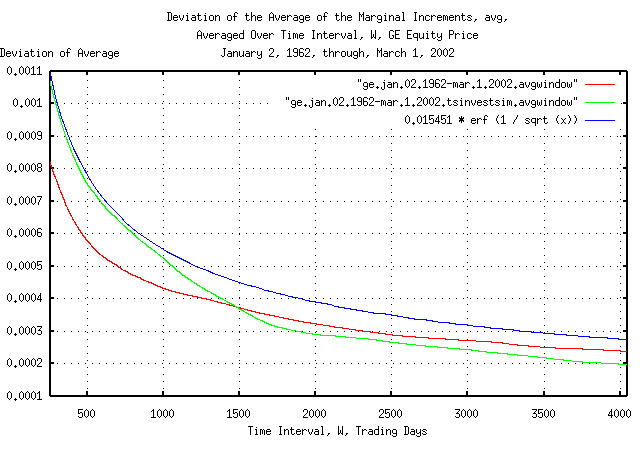Figure III

Figure III is a plot of the cumulative probability of the duration of the increases and decreases in the average of the marginal increments, `avg`, of GE's equity price, and its simulation, with the theoretical value, ```0.015451 * erf (1 / sqrt (t))```. The frequency of the durations of the increases and decreases of 253 trading days, (about a calendar year,) to 4,048 trading days, (about 16 calendar years,) are shown. Figure III is the same as Figure I, but shows only the frequency of long durations of increases and decreases for clarity. Since the data set size consisted of only 10,113 trading days, the empirical cumulative probability is smaller than the theoretical value by ```0.015451 * erf (1 / sqrt (10113)) = 0.015451 * 0.011968 = 0.000184917568``` at 4,048 trading days.

Figures I, II, and III, are the cumulative probability of the duration of the increases and decreases in the average of the marginal increments, `avg`, of GE's equity price. For example, at the end of any 500 trading day interval, (about 2 calendar years,) the average of the marginal increments would be within about, plus, or minus, `0.0008` of its mean value of `0.000507` for one standard deviation, about 68%, of the time.

The cumulative probability of the duration of the increases and decreases in the deviation of the marginal increments, `rms`, of GE's equity price:

``````

#!/bin/sh

interval=1

while [ \$interval -le 4048 ]
do

tsmath -l ge.jan.02.1962-mar.1.2002 | tslsq -o | \
tsderivative | tsrmswindow -w \$interval | tsmath -s 0.015451 | tsrms -p >> \
ge.jan.02.1962-mar.1.2002.rmswindow

tsmath -l ge.jan.02.1962-mar.1.2002.tsinvestsim | tslsq -o | \
tsderivative | tsrmswindow -w \$interval | tsmath -s 0.015451 | tsrms -p >> \
ge.jan.02.1962-mar.1.2002.tsinvestsim.rmswindow

interval=`expr \$interval + 1`

done

``````

which uses the `tsrmswindow` program to calculate the deviation of the marginal increments over the time interval, `interval`, for all intervals from `1` to `4048`, the `tsmath` program to subtract the mean value of the deviation of the marginal increments, `rms`, and the `tsrms` program to calculate the deviation of the deviation for GE's equity price.Figure IV

Figure IV is a plot of the cumulative probability of the duration of the increases and decreases in the deviation of the marginal increments, `rms`, of GE's equity price, and its simulation, with the theoretical value, ```0.015451 * erf (1 / sqrt (t))```. The frequency of the durations of the increases and decreases of up to 4,048 trading days, (about 16 calendar years,) are shown.

The apparent discrepancy between the empirical and theoretical values of the cumulative probability of the duration of the increases and decreases in the deviation of the marginal increments is an artifact of the interaction between the windowing construct used for measuring the deviation over time intervals and a 13 year cyclic clustering phenomena in the time series. See Appendix I for particulars.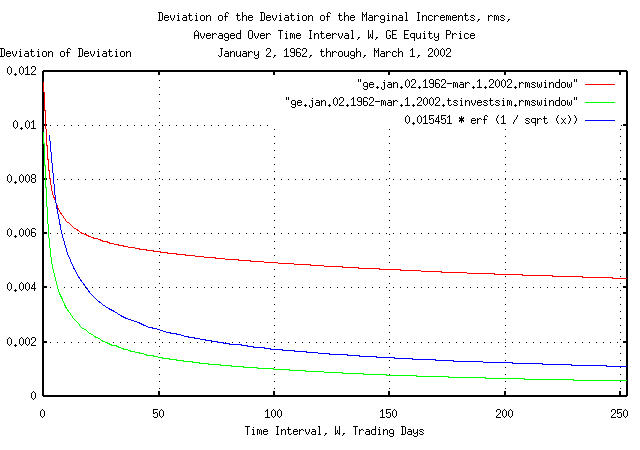Figure V

Figure V is a plot of the cumulative probability of the duration of the increases and decreases in the deviation of the marginal increments, `rms`, of GE's equity price, and its simulation, with the theoretical value, ```0.015451 * erf (1 / sqrt (t))```. The frequency of the durations of the increases and decreases of up to 253 trading days, (about a calendar year,) are shown. Figure V the same as Figure IV, but shows only the frequency of short durations of increases and decreases for clarity.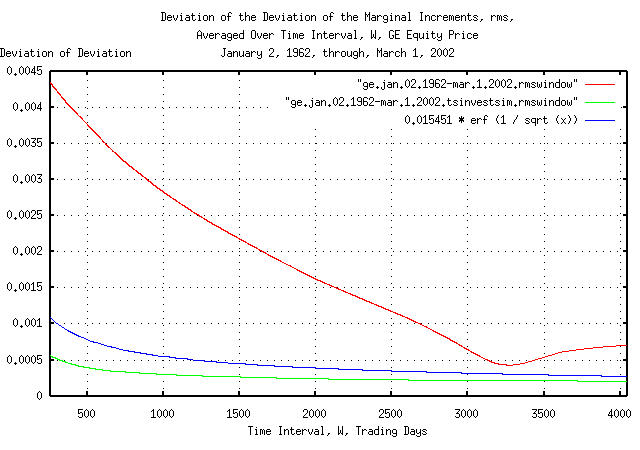Figure VI

Figure VI is a plot of the cumulative probability of the duration of the increases and decreases in the deviation of the marginal increments, `rms`, of GE's equity price, and its simulation, with the theoretical value, ```0.015451 * erf (1 / sqrt (t))```. The frequency of the durations of the increases and decreases of 253 trading days, (about a calendar year,) to 4,048 trading days, (about 16 calendar years,) are shown. Figure VI is the same as Figure IV, but shows only the frequency of long durations of increases and decreases for clarity. Since the data set size consisted of only 10,113 trading days, the empirical cumulative probability is smaller than the theoretical value by ```0.015451 * erf (1 / sqrt (10113)) = 0.015451 * 0.011968 = 0.000184917568``` at 4,048 trading days.

Figures IV, V, and VI, are the cumulative probability of the duration of the increases and decreases in the deviation of the marginal increments, `rms`, of GE's equity price. For example, at the end of any 500 trading day interval, (about 2 calendar years,) the deviation of the marginal increments would be within about, plus, or minus, `0.00075` of its mean value of `0.015451` for one standard deviation, about 68%, of the time.

The cumulative probability of the duration of the increases and decreases in the likelihood of an up movement in the marginal increments, `P`, of GE's equity price:

``````

#!/bin/sh

interval=1

while [ \$interval -le 4048 ]
do

tsshannonwindow -a -w \$interval ge.jan.02.1962-mar.1.2002 | \
tsmath -s 0.51640670507 | tsrms -p >> ge.jan.02.1962-mar.1.2002.Pwindow

tsshannonwindow -a -w \$interval ge.jan.02.1962-mar.1.2002.tsinvestsim | \
tsmath -s 0.51640670507 | tsrms -p >> ge.jan.02.1962-mar.1.2002.tsinvestsim.Pwindow

interval=`expr \$interval + 1`

done

``````

which uses the `tsshannonwindow` program to calculate the likelihood of an up movement, `P`, of the marginal increments over the time interval, `interval`, for all intervals from `1` to `4048`, the `tsmath` program to subtract the mean value of the likelihood of an up movement of the marginal increments, `P`, and the `tsrms` program to calculate the deviation of the likelihood of an up movement of the marginal increments.Figure VII

Figure VII is a plot of the cumulative probability of the duration of the increases and decreases in the likelihood of an up movement in the marginal increments, `P`, of GE's equity price, and its simulation, with the theoretical value, `0.51640670507 * erf (1 / sqrt (t))`. The frequency of the durations of the increases and decreases of up to 4,048 trading days, (about 16 calendar years,) are shown.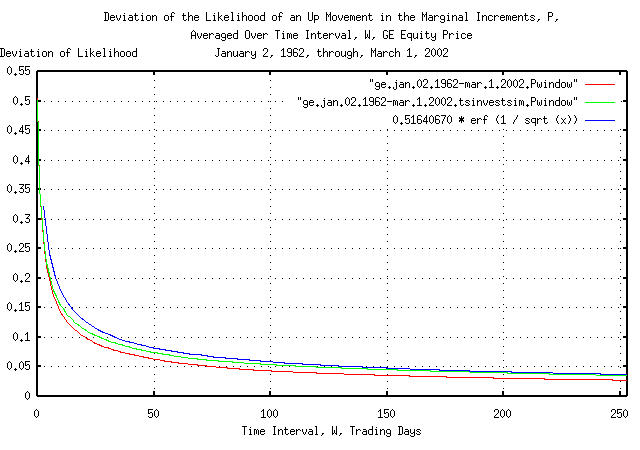Figure VIII

Figure VIII is a plot of the cumulative probability of the duration of the increases and decreases in the likelihood of an up movement in the marginal increments, `P`, of GE's equity price, and its simulation, with the theoretical value, `0.51640670507 * erf (1 / sqrt (t))`. The frequency of the durations of the increases and decreases of up to 253 trading days, (about a calendar year,) are shown. Figure VIII the same as Figure VII, but shows only the frequency of short durations of increases and decreases for clarity.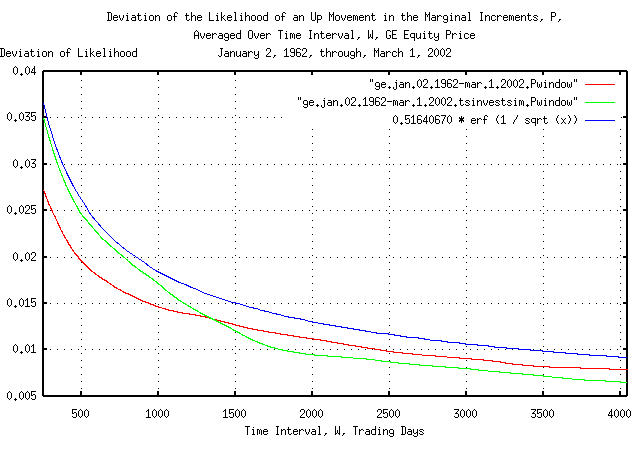Figure IX

Figure IX is a plot of the cumulative probability of the duration of the increases and decreases in the likelihood of an up movement in the marginal increments, `P`, of GE's equity price, and its simulation, with the theoretical value, `0.51640670507 * erf (1 / sqrt (t))`. The frequency of the durations of the increases and decreases of 253 trading days, (about a calendar year,) to 4,048 trading days, (about 16 calendar years,) are shown. Figure IX is the same as Figure VII, but shows only the frequency of long durations of increases and decreases for clarity. Since the data set size consisted of only 10,113 trading days, the empirical cumulative probability is smaller than the theoretical value by ```0.51640670507 * erf (1 / sqrt (10113)) = 0.51640670507 * 0.011968 = 0.00618035545``` at 4,048 trading days.

Figures VII, VIII, and IX, are the cumulative probability of the duration of the increases and decreases in the likelihood of an up movement in the marginal increments, `P`, of GE's equity price. For example, at the end of any 500 trading day interval, (about 2 calendar years,) the likelihood of an up movement in the marginal increments would be within about, plus, or minus, `0.025` of its mean value of `0.51640670507` for one standard deviation, about 68%, of the time.

The cumulative probability of the duration of the increases and decreases in the gain of the marginal increments, `g`, of GE's equity price:

``````

#!/bin/sh

interval=1

while [ \$interval -le 4048 ]
do

tsgainwindow -w \$interval ge.jan.02.1962-mar.1.2002 | \
tsmath -s 1.00038773455 | tsrms -p >> ge.jan.02.1962-mar.1.2002.gwindow

tsgainwindow -w \$interval ge.jan.02.1962-mar.1.2002.tsinvestsim | \
tsmath -s 1.00038773455 | tsrms -p >> ge.jan.02.1962-mar.1.2002.tsinvestsim.gwindow

interval=`expr \$interval + 1`

done

``````

which uses the `tsgainwindow` program to calculate the gain, `g`, of the marginal increments over the time interval, `interval`, for all intervals from `1` to `4048`, the `tsmath` program to subtract the mean value of the gain of the marginal increments, `g`, and the `tsrms` program to calculate the deviation of the gain of the marginal increments.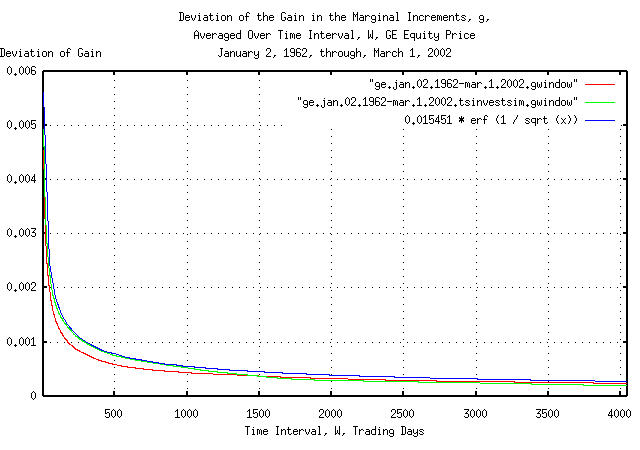Figure X

Figure X is a plot of the cumulative probability of the duration of the increases and decreases in the gain of the marginal increments, `g`, of GE's equity price, and its simulation, with the theoretical value, ```0.015451 * erf (1 / sqrt (t))```. The frequency of the durations of the increases and decreases of up to 4,048 trading days, (about 16 calendar years,) are shown.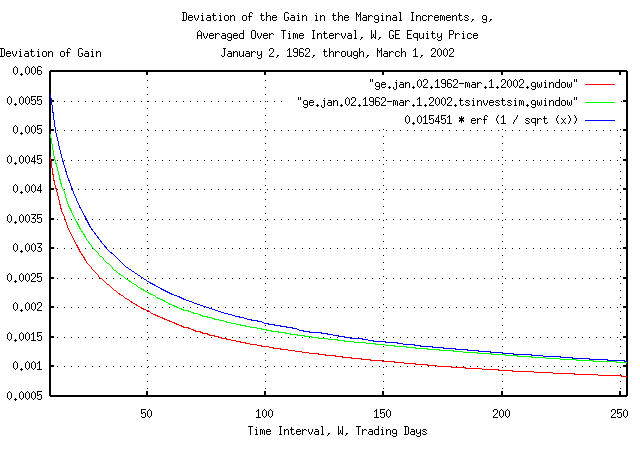Figure XI

Figure XI is a plot of the cumulative probability of the duration of the increases and decreases in the gain of the marginal increments, `g`, of GE's equity price, and its simulation, with the theoretical value, ```0.015451 * erf (1 / sqrt (t))```. The frequency of the durations of the increases and decreases of up to 253 trading days, (about a calendar year,) are shown. Figure XI the same as Figure X, but shows only the frequency of short durations of increases and decreases for clarity.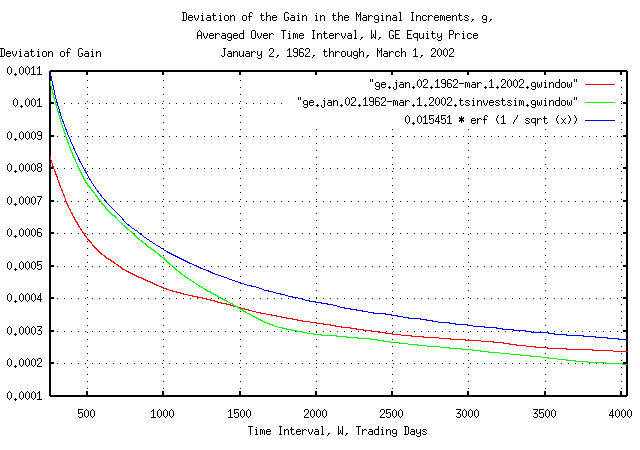Figure XII

Figure XII is a plot of the cumulative probability of the duration of the increases and decreases in the gain of the marginal increments, `g`, of GE's equity price, and its simulation, with the theoretical value, ```0.015451 * erf (1 / sqrt (t))```. The frequency of the durations of the increases and decreases of 253 trading days, (about a calendar year,) to 4,048 trading days, (about 16 calendar years,) are shown. Figure XII is the same as Figure X, but shows only the frequency of long durations of increases and decreases for clarity. Since the data set size consisted of only 10,113 trading days, the empirical cumulative probability is smaller than the theoretical value by ```0.015451 * erf (1 / sqrt (10113)) = 0.015451 * 0.011968 = 0.000184917568``` at 4,048 trading days.

Figures X, XI, and XII, are the cumulative probability of the duration of the increases and decreases in the gain of the marginal increments, `g`, of GE's equity price. For example, at the end of any 500 trading day interval, (about 2 calendar years,) the gain of the marginal increments would be within about, plus, or minus, `0.0011` of its mean value of `1.00038773455` for one standard deviation, about 68%, of the time.

 As a side bar, none other than Sir Isacc Newton, referenced in Harvard Magazine's The Damn'd South Sea, was foiled by the dynamics of speculative markets-as were many more recently in the dot-com, (which came to be called the dot-bomb,) market. An equity increasing in price, generously, for two years is no indication that the good fortune will continue. About 16%, one deviation, of the time, it would be expected that GE's equity gain, `g`, would be greater than about ```1.00038773455 + 0.0011 = 1.00148773455``` per day averaged over any 2 calendar year interval-meaning increasing in value by a factor of at least ```(2 * 253)^1.00148773455 = 2.12173522976``` in the 2 calendar years of 253 trading days in a calendar year. That's the good news. The bad news is that at 1,012 trading days, (or about 2 years later,) the chances that GE's equity price would still be maintaining the factor of 2 growth in value would be about ```0.0011 / 0.0005 = 2.2``` deviations, which is about one chance in 72.

### Example II, The Annual Fluctuations of Equities

How much would a typical equity's price on the US exchanges be expected to fluctuate in a year?

From Section III, Equation (3.1):
``````

rms * sqrt (t) .....................................(3.1)

``````

which is a formula for the deviation of the magnitude of bubbles for the Brownian motion/random walk equivalent of equity prices. It is, also, the range, `R`, often used in Range/Scale, (Hurst,) analysis.

Converting to log-normal characteristics, the maximum, and minimum, respectively would be:

``````

+rms * sqrt (t)
V0 * e                ..............................(4.1)

-rms * sqrt (t)
V0 * e                ..............................(4.2)

``````

where `rms` is the deviation of the marginal increments in an equity's price, `v0` is the starting value of an equity price, and `t`, is some time in the future.

The median value for the daily marginal increments in an equity price on the US exchanges is about ```rms = 0.02```, so for `t = 253` trading days in a calendar year, a typical equity's minimum and maximum price would be:

``````

+0.02 * sqrt (253)
V0 * e                   = 1.37454047383 ...........(4.3a)

-0.02 * sqrt (253)
V0 * e                   = 0.727515864 .............(4.4a)

``````

A typical equity's price maximum, divided by its minimum, in a year, will be `1.8893615142`, or about a factor of `2`, where typical means a standard deviation, (i.e., about 68% will be less, 32%, more.)

Its interesting, because from "Reuter", April 27, 1997, Peter Lynch was quoted as saying:

... Stocks are volatile ... the average stock listed on the New York Stock Exchange fluctuates 50 percent between its annual high and low ...

### Example III, The Durability of US Public Companies

Although non-linear high entropy economic systems typically exhibit long term exponential growth, that does not mean they can not go bust. An interesting question is how long a public company, on average, is listed on an exchange.

It is a difficult analytical problem because of the discontinuities; companies have different initial public offering equity prices, there are splits which effectively reduce the IPO price, and when a company's equity price falls below one dollar, it is de-listed. Not to mention that companies get de-listed when they are acquired, too.

However, some work on http://www.google.com/ indicated that after adjusting for splits, a reasonable estimate for the median, (half more, half less,) value of the IPO price for US equities in the Twentieth Century was about two dollars.

For the Twentieth Century, the median value, (half more, half less,) of the average and deviation of the daily marginal increments in equity prices for those listed on the US exchanges was about `avg = 0.0004`, and, ```rms = 0.02```, respectively.

 As an interesting side bar, from Section I, Equation (1.24), and, Equation (1.18): `````` avg 0.0004 --- + 1 ------ + 1 rms 0.02 P = ------- = ------------ = 0.51 ..................(1.24) 2 2 `````` `````` rms = 2 P - 1 = 0.02 = 2 * 0.51 - 1 = 0.02 .........(1.18) `````` meaning that most, (actually, the median,) of the equities of US public companies in the Twentieth Century grew maximally optimal.

The following `tsinvestsim` program file, `exchange`, was made for 500 companies:

``````

0, p = 0.51, i = 2
1, p = 0.51, i = 2
.
.
.
498, p = 0.51, i = 2
499, p = 0.51, i = 2

``````

where the company names are ```0, 1, ... 498, 499``` and all the company's stocks are statistically identical, i.e., they all start at an IPO price of two dollars, and are maximally optimal with `avg = 0.0004`, `rms = 0.02`, and ```P = 0.51```.

The C source code to the `tsinvestsim` program had to be modified for the simulation so that whenever a company's equity value dropped below a dollar, it had to be de-listed. The following construct was added to the `tsinvestsim` C source code, version 1.3, dated 2001/12/07 10:05:09, at line 588:

``````

if (sum < (double) 1.0) /* stock's value less than a buck? */
{
sum = (double) 0.0; /* yes, de-list the stock */
stock->sum = (double) 0.0;
stock->f = (double) 0.0;
}

``````

which, if an equity's value is ever less than one dollar, set's it to zero, forever. A simulation of ```5,566 = 22 * 253``` trading days, or 22 calendar years of 253 trading days in a calendar year:

``````

./tsinvestsim exchange 5566 > result

``````

was run, and the last 500 records of the output file, `result`, searched for equities with zero value. There were `206` out of the `500`, or `41.2%` of the companies had been de-listed in the 22 calendar year simulation.

The simulation duration of 22 calendar years was chosen because during the Twentieth Century, the median time a company was listed on the US exchanges was 22 years. Half were listed for less, half more. The `41.2%` simulation number is within about 20% of the empirical number. (Although `41%` of the companies in the simulation had failed by 22 years, one had an equity price of `\$1333.721702`, and the median value of the 500 equities was `\$3.207836`, compared with a calculated value of `\$3.04430985883` from Section I, Equation (1.20). Almost a perfect log-normal distribution.)

 As a side bar, this is an intuitive result. Using round numbers, during the Twentieth Century a company had a 50% chance of being de-listed every 20 years, or so. So, at the end of 20 years, 50% of the companies would remain. At the end of 40 years, 25% would remain, and at the end of 60, 12.5%, and 6.25% at 80 years, and after a century, 3.125%. There are 30 companies in the DJIA. Of the original that were in the DJIA in 1900, it would be expected that that `30 * 0.03125 = 0.9375`, or about one, would be listed through the century to 2000. There was exactly one; GE. (Which is why the GE equity price time series was chosen for analysis-it has the largest data set size.) The exponential characteristics of Equation (1.20) would tend to imply that a company's equity value would increase forever. That would be true if ```avg = rms``` and `P = 1` in Equation (1.24). But they aren't, making the destiny of all companies, given enough time, the same.

The `tsinvestsim` C source code is available in the tsinvest distribution available from the NtropiX site.

 As a footnote addendum to this section, (October 19, 2010,) the same is true for the hedge fund industry, too. According to The Quants: How a New Breed of Math Whizzes Conquered Wall Street and Nearly Destroyed It, Scott Patterson, Crown Business, a division of Random House, Inc., New York, New York, 2010, ISBN 978-0-307-45337-2, (which is highly recommended reading,) hedge funds return, on average, about 20% a year in good times, (to a one-time maximum as high as 80%.) This means the average and deviation of the daily marginal increments of a typical hedge fund would be about `avg = 0.0016`, and, `rms = 0.04`, respectively. Or, the `tsinvestsim` program file, `fund`, would be, (for an industry of 500 hedge funds): `````` 0, p = 0.52, i = 2 1, p = 0.52, i = 2 . . . 498, p = 0.52, i = 2 499, p = 0.52, i = 2 `````` where the hedge fund names are ```0, 1, ... 498, 499``` and all the fund's are statistically identical, i.e., they all start at an value price of two dollars, and are maximally optimal with ```avg = 0.0016```, ```rms = 0.04```, and ```P = 0.52```. A simulation of ```5,566 = 22 * 253``` business days, or 22 calendar years of 253 business days in a calendar year: `````` ./tsinvestsim fund 5566 > result `````` yields 47.29%, (`236` out of `500`,) of the hedge funds went broke within 22 years, with the value of the smallest survivor \$2.20, and the value of the largest survivor, \$6,564,988.86! The hedge fund industry will have a major "crash" about every 20 years, (the number of fund failures will actually tend to cluster in times of uncertainty,) where about half the hedge funds go out of business. This statistic is supported by the evidence, too. According to A Brief History Of The Hedge Fund by James E. McWhinney, the hedge fund was invented in 1949, with the first crash in 1969-1974, the second 1997-2000, and the third, 2009-2010, for an average of 20 years between hedge fund industry crashes, which is very close to the simulated value, and nearly identical to the durability of US public companies, (See: "The Real Jobs Machine," Robert J. Samuelson, Newsweek, October 11, 2010, pp. 28, which states, "A company founded today has an 80% chance of disappearing over the next quarter century, reports a study by Dane Stangler and Paul Kedrosky of the Kauffman foundation.") This is in reasonable agreement with the theoretical simulations, above, too. Panics, (happening randomly,) on average about every twenty years are an investing inevitability. And, that is true for any non-linear high entropy economic system, including recessions in the GDP, (see: List of recessions in the United States, where the recessions lasting more than one year since the Great Depression are, 1929, 1937, 1973, 1980, and, 2009, which happened, on average, every 20 years,) currency exchange rates, etc. The "mechanism" of the panics is a characteristic of the random process itself-an economic system will experience exponential growth, and sooner or later, the random risks will cluster, overwhelming the exponential growth, and the growth will turn negative for a substantial time-making investing a "deal with the devil." It is similar to a pyramid scheme, where the strategy is to be first-in and first-out. Note the consequences: if one doesn't play, one can't win; but if one plays long enough, one looses. But, doesn't this contradict the paradigm of John Kelly's Kelly criterion? The answer is no. Note that all of the simulations in this section required a "structural" addition to the `tsinvestsim` program, i.e., if an equity's value drops below one dollar, it is removed from the exchange, (and probably goes into receivership,) or, if a hedge fund loses half of its original value, margin calls will force it to liquidate. However, theoretically, given enough time, the equity, or hedge fund, would make a comeback, if there were not structural limitations on how much an investment can lose before it is forced out of business.

As a qualitative approximation model, (i.e., simplified conceptual interpretation for Brownian motion fractals, using the stochastic calculus, which is not adequate for quantitative analysis,) the economic system, (equity value, GDP, currency exchange rate, etc.) is always increasing or decreasing, randomly, (i.e., "bubbles," always increasing or decreasing, randomly.) The probability of a bubble lasting at least `t` many years is, approximately, `1 / sqrt (t)`. For example, the probability of a positive, (or negative,) parts of the, (Juglar,) business cycle lasting at least 4 years is ```1 / sqrt (4) = 50%```, (half of the positive or negative parts of the business cycles would last less, half more); the chances of a business cycle "bubble" lasting at least twenty five years would be `1 / sqrt (25) = 20%`, and so on. How big the "bubble" will be, in one year, (if it lasts that long,) is probabilistic, too, with a standard deviation of approximately ```0.3 * sqrt (t)``` for many/most economic systems. For example, 68% of the time, the economic system movement will be less than +/- 30% in one year; in four years, less than +/- 60%.

Or, modeling the five positive or negative parts of the business cycles, (each lasting a median of four years, half lasting more, half lasting less,) over an typical 20 year period, one of the business cycles, on average, would have a negative economic system movement of at least a standard deviation, (assuming one out of five is 20% of the time, and is about equal to 16%, which is the the value of the standard deviation-not being precise, this is a simple "thought" model, technically, with a 50% chance,) or a movement of about 60%, which is very close to the 50% loss simulated, above.

Note the simplicity of the economic model-which only assumes that unpredictable things happen to an an economic system, (economy, equity value, etc.,) in a random fashion, which moves the economic system from its current value or state. Once that is assumed, the durability of the economic system is defined by the stochastic calculus.

### Appendix I, A Useful Approximation

An analysis of the deviation of the marginal increments of GE's equity price indicates that the deviation is not stable:

``````

tsmath -l ge.jan.02.1962-mar.1.2002 | tslsq -o | \
tsderivative | tsrmswindow -w 50 > ge.jan.02.1962-mar.1.2002.rmswindow-50

``````

where the `tsrmswindow` program is used to calculate the deviation of the marginal increments over a moving window of `50` trading days for GE's equity price.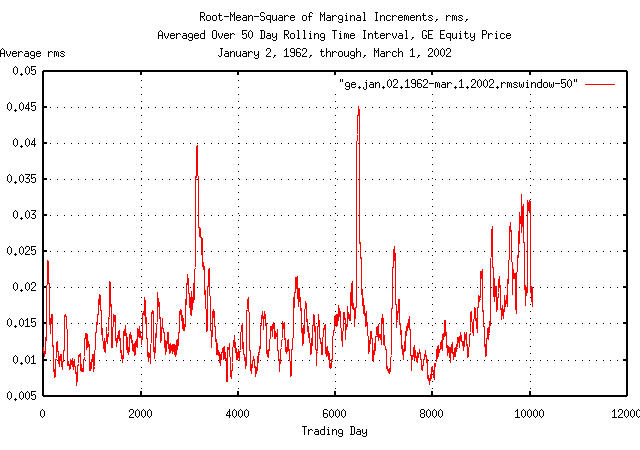Figure XIII

Figure XIII is a plot of the deviation, (root-mean-square,) of the marginal increments, `rms`, of GE's equity price, using a `50` trading day moving window for the calculation of the deviation.

Apparently, there is cyclic phenomena occurring about every `3,300` trading days, (about 13 calendar years,) causing the calculation of the deviation of the deviation of the marginal increments of GE's equity price to be too large everywhere except the dips at `3,300` days in Figure IV and Figure VI. The phonomena is quite complex:

Marginal Increment Date

0.066667

1962-07-02

-0.089744

1974-08-23

0.070423

1974-08-26

-0.065789

1974-09-04

-0.066667

1974-09-09

0.065574

1974-09-16

0.088235

1974-10-09

-0.174757

1987-10-19

0.082353

1987-10-20

-0.068293

1987-10-22

-0.084656

1987-10-26

0.064426

1987-11-05

-0.083333

1988-01-08

-0.106904

2001-09-17

-0.065502

2001-09-20

0.124352

2001-09-24

TABLE I.

Note that:

1. The values are quite large-for example, on 1987-10-19, GE's equity price suffered a `17.4757%` drop in value; a ```0.174757 / 0.015451 = 11.3104006213``` deviation incident, which has a probability of happening that is indistinguishable from zero-yet it did happen.

2. The large excursions in high volatility tend to cluster, usually occuring for a few weeks to a few months.

3. They happen infrequently, and possibly by serendipity, are cyclic in nature.

The mechanisms creating periods of high volatility are not clearly understood, and create substantial leptokurtosis in the frequency distribution of the marginal increments as shown in Section I, Figure II. The prevailing explanations are:

• They are created by structural phenomena-they usually occur in the fourth calendar quarter when taxation requirements are addressed by investors.

• Or, the probability distribution is more complex than is generally thought-tending to be produced by a non-linear mechanism, having Cauchy distribution like characteristics.

• Or, possibly, they are the result of complex non-linear dynamical system characteristics, which can phase lock to structural phenomena.

The high volatility days that are inconsistent with the statistical model used occurred in 16 out of the 10,113 days between January 2, 1962, through, March 2, 2002. There are several options available to address the discrepancies created:

1. Remove the 16 offenders from the data set.

2. Reduce their values to be consistent with the model.

3. Shuffle the 16 offenders around in the data such that their effects do not cluster, and are not synchronous with any window size used in the analysis.

The latter is preferred since much of the analytical data will remain the same, (i.e., the average, deviation, and gain, of the marginal increments, `avg`, `rms`, and, `g`, respectively, will not change.)

A software strategy to shuffle the marginal increments in a time series would be to generate a new first column in the data set's tabular time series consisting of random numbers, sort on the random numbers, and then remove the column, leaving the original data set in a shuffled random order:

``````

tsgaussian 10113 > random
tsfraction ge.jan.02.1962-mar.1.2002 > fraction
paste random fraction | sort -n | \
cut -f2 > ge.jan.02.1962-mar.1.2002.shuffled

while [ \$interval -le 6325 ]
do
tsmath -s 0.000507 ge.jan.02.1962-mar.1.2002.shuffled | \
tsrmswindow -w \$interval | tsmath -s 0.015451 | \
tsrms -p >> ge.jan.02.1962-mar.1.2002.rmswindow.shuffled
done

``````

where the `tsgaussian` program is used to generate a file, `random`, consisting of 10113 random numbers. The `tsfraction` program is used to make a file, `fraction`, of the marginal increments of GE's equity price. The two files are tabularized into a single file with two columns using the Unix paste command, numerically sorted on the first column of random numbers using the Unix sort command, and then the first column removed, using the Unix cut command, leaving only the marginal increments of GE's equity price, in random order. The remainder of the proceedure is identical to the process that created the graphs in Figure IV and Figure VI.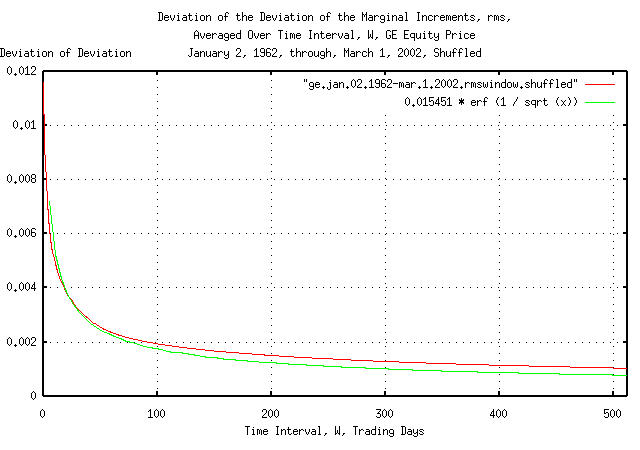Figure XIV

Figure XIV is a plot of the cumulative probability of the duration of the increases and decreases in the deviation of the shuffled marginal increments, `rms`, of GE's equity price with the theoretical value, ```0.015451 * erf (1 / sqrt (t))```. The frequency of the durations of the increases and decreases of up to 506 trading days, (about two calendar years,) are shown.

 As a side bar, many quantitative analyst-sometimes called financial engineers-place more importance on the deviation of the marginal increments of time series than any other variable; including asset appreciation. The deviation is a metric of risk. Peter Lynch is accredited with the proverb "making money on Wall Street is easy-keeping it is the hard part." A very small chance of something happening, given enough time, will happen, and that's why its hard. Mitigating risk is how one keeps the money. But we have just seen how difficult it is to get accurate metrics on risk. Most market technicians use a set of cross-checks on the metrics of risk. For example, there were 10,113 trading days represented in the time series for GE's equity price, between January 2, 1962, and March 1, 2002. That means that the largest daily movement would be a `3.92` sigma event, since a 1 in 10,000 chance is `3.92` deviations. The largest movement occurred on October 19, 1987, when GE's equity price dropped `17.4757%`, which would mean that the deviation of the marginal increments should be around `0.174757 / 3.92 = 0.0445808673`, which is about a factor of three times `0.015451` which was measured in the analysis, based on only one observation in the tails of the distribution. Its an important concept since statistical anomalies, i.e., those measurements that do not fit a dogmatic model, are often simply discarded in the pseudo-sciences. But what our cross-check is telling us is that our risk of extreme movements in GE's equity price is about 3 times what we think it is! Continuing on, there should be 27 out of 10,000 `3` sigma events-or the 27 next largest movements, (all you have to do is numerically sort the time series of the marginal increments to get them,) and the value of the 27'th is `0.065789`, or the deviation of the marginal increments based on the the 3 sigma tail events would be ```0.065789 / 3 = 0.0219296667```. For 2 sigma events, there should be 460 out of 10,000-and the 460'th largest movement was `0.031915`, meaning that based on 2 sigma events, the deviation of the marginal increments would be `0.031915 / 2 = 0.0159575`, which is reasonably close to `0.015451`, the value measured using the daily returns. Our conclusion is that the model seems to hold fairly well for slightly past 2 sigma events-possibly even to 3 sigma-but beyond 3 sigma, and into 4 sigma, we have to increase our risk assessment metric for an investment in GE's equity by at least a factor of 3. In financial engineering, the excess risk of loss do to the tail events of the distribution is called Expected Tail Loss, `ETL`, which is also known as expected shortfall, or, worst conditional expectation. It is usually estimated as a probability-weighted average of tail losses above what would be expected in a normal/Gaussian distribution of the marginal increments, (with a deviation = `rms`,) in an equity's price-which is also known in financial engineering as Value-at-Risk, `VaR`. For example, the negative tails in TABLE I, above, total `-0.805645` for the `10,113` trading days, so the risk would be increased by about ```0.805645 / 10113 = 0.0000796642935``` per day, meaning that for a calendar year of `253` trading days, the risk would increase by about `0.02`, or about double from `0.015` to `0.035`. (Note that this is the value of negative short term `ETL`-catastrophic loss that does not fit the model in a calendar year; what we usually worry about. There were positive tails, too-the total excess above-two-sigma movements in TABLE I is `-0.310282`, meaning the `ETL` estimate calculated above is a factor of `2.5` too large for long term projections.)

Why the discrepancy?

``````

tsmath -l ge.jan.02.1962-mar.1.2002 | tslsq -o | tsroot -l | \
tsscalederivative > ge.jan.02.1962-mar.1.2002
tsinvestsim -n 1000 sim 10113 | tsmath -l | tslsq -o | tsroot -l | \
tsscalederivative > tsinvestsim.jan.02.1962-mar.1.2002

``````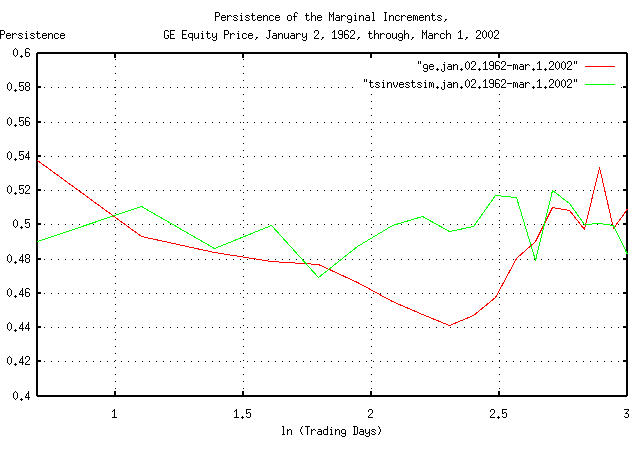Figure XIV

Figure XIV is a plot of the persistence in the daily returns of GE's equity price. Note that there is significant short term persistence, (about 0.54,) meaning that there is a 54% chance of GE's equity price doing tomorrow, what it did today. This is probably a short term inefficiency, (in the sense of the EMH, the Efficient Market Hypothesis.) However, there is also an indication of a period of anti-persistence of about ```e^2.7 = 15``` days, too-which is probably related to structural issues, (for example, a high probability of a cyclic downward spike every year would do this; probably near late October, when mutual funds account for capital and income gains for tax reasons, which can be seen in TABLE I.)

This type of persistence, (actually a cyclic issue,) would increase the likelihood of large swings, and they would tend to cluster. In addition, the leptokurtosis shown in the analysis of GE's equity price in Section I, would tend to increase the relative number of large movements in price.

### Appendix II, A Useful Methodology

It would be advantageous to develop a methodology to quantify the persistence/leptokurosis/root inefficiencies of a time series.

In the following analysis, the historical time series of the DJIA, NASDAQ, and S&P500 indices through December 5, 2002 was obtained from Yahoo!'s database of equity Historical Prices, (ticker symbols ^DJI, ^IXIC, and, ^SPC, respectively,) in csv format-which was converted to a Unix database format using the `csv2tsinvest` program. The converted filename were `djia1900-2002`, `nasdaq1984-2002`, and `sp1928-2002`, respectively.

``````

tsmath -l djia1900-2002 | tsroot -l > djia.jan.02.1900-dec.05.2002
tsmath -l nasdaq1984-2002 | tsroot -l > nasdaq.oct.11.1984-dec.05.2002
tsmath -l sp1928-2002 | tsroot -l > sp.jan.03.1928-dec.05.2002
tsinvestsim -n 1000 sim 100000 | tsmath -l | tsroot -l > simulation.100000

``````

where the file `sim` contains:

``````

dummy, p = 0.51

``````

and is a simulation, for comparison, where the average of the marginal increments, `avg = 0.0004`, and the root-mean-square of the marginal increments, ```rms = 0.02```, giving a likelihood of an up movement, `P`, of `0.51`-the dynamic characteristics of a typical equity's value on the US exchanges.

Using the `tslsq` to calculate the linear least squares fit approximation of the first `e^3 = 20.0855369232` trading days, (approximately one calendar month):

``````

egrep '^[0-2]\.' djia.jan.02.1900-dec.05.2002 | tslsq -p
-4.915572 + 0.539041t
egrep '^[0-2]\.' nasdaq.oct.11.1984-dec.05.2002 | tslsq -p
-4.665253 + 0.558219t
egrep '^[0-2]\.' sp.jan.03.1928-dec.05.2002 | tslsq -p
-4.884043 + 0.534678t
egrep '^[0-2]\.' simulation.100000 | tslsq -p
-4.138124 + 0.498988t

``````

and plotting for ```e^4.14709512761 = 63.25``` trading days, (about a calendar quarter):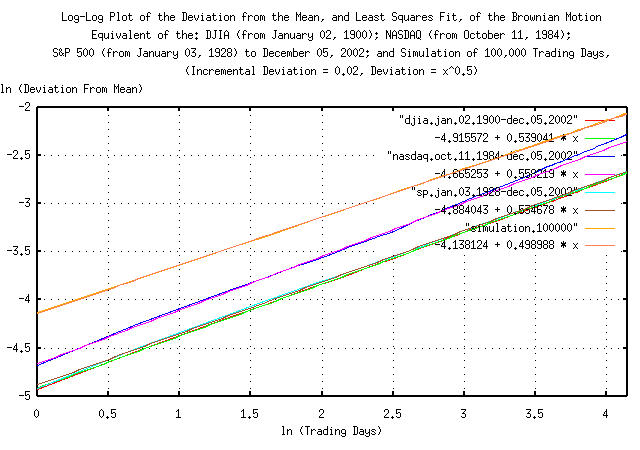Figure XV

Figure XV is a Log-Log plot of the Deviation from the mean, and least squares fit, of the Brownian motion/random walk equivalent of the DJIA, (from January 2, 1900, through, December 5, 2002,) the NASDAQ, (from October 11, 1984, through, December 5, 2002,) the S&P500, (from January 3, 1928, through, December 5, 2002,) and a 100,000 trading day simulation of a typical equity's value from the US exchanges. The slope of the lines in the graph is the root of the deviation-i.e., for the simulation, it would be 0.5, (meaning square root,) or root mean square mathematics should be used, (implying infinite entropy, uncertainty, or unpredictability.)

Of interest is the linearity of the empirical metrics-at least for a calendar quarter of daily closes.

Considering only the dynamics, the deviation of the indices' Brownian motion/random walk equivalent for the DJIA, NASDAQ, and S&P500 from their mean values would be:

``````

(e^-4.915572) * (t^0.539041) = 0.00733152306 * t^0.539041
(e^-4.665253) * (t^0.558219) = 0.00941686546 * t^0.558219
(e^-4.884043) * (t^0.534678) = 0.00756636131 * t^0.534678

``````

respectively, compared with the values derived using the `tsfraction` and `tsrms` programs:

``````

0.011028 * t^0.5
0.014448 * t^0.5
0.011367 * t^0.5

``````

respectively. (Note that the exponents represent a system with a Hausdorff fractal dimension following a power law relationship-for a Gaussian/normal distribution of the marginal increments, the relationship is `1 / 0.5 = 2`; larger than `0.5` represents a time series with marginal increments that have a deviation that is non-stable, or diverging-a Pareto-Levy distribution-which does have implications; see: Lessons of the Fall: A Stunning Collapse and Weighty Morals to the Story.)

And, how much difference is there between the actual, empirical theoretic, and standard deviation approximation for the DJIA, NASDAQ, and S&P500? As an informal, (the deviation is not stable, and diverges,) pictographic interpretation:

``````

tsmath -l djia1900-2002 | tsroot > djia.jan.02.1900-dec.05.2002.a
tsmath -l nasdaq1984-2002 | tsroot > nasdaq.oct.11.1984-dec.05.2002.a
tsmath -l sp1928-2002 | tsroot > sp.jan.03.1928-dec.05.2002.a

``````

and plotting:Figure XVI

Figure XVI is a plot of the "deviation" from the mean, least squares fit of variables, and standard deviation approximation of the Brownian motion/random walk equivalent of the DJIA, (from January 2, 1900, through, December 5, 2002,) the NASDAQ, (from October 11, 1984, through, December 5, 2002,) the S&P500, (from January 3, 1928, through, December 5, 2002,) for 512 trading days, (about two calendar years.) The top three graphs are the NASDAQ, and the DJIA and S&P500 are the bottom six, or approximately a +/- 10% error at two calendar years.

Remember, as a mathematical expediency, the analysis uses the Brownian motion/random walk equivalent of the time series for the indices-to convert back to a log-normal distribution, find the median values, and multiply the median values by the exponentiated values in Figure XVI.

```
--

John Conover, john@email.johncon.com, http://www.johncon.com/

```